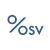0
Fixed

# Problems with Sales Growth on Portfolio Report Views

Rob 4 years ago updated 4 years ago

There seems to be some problems with the data showing sales growth in the portfolio report views. I created a custom report and wanted to show growth values (3 year and 10 year). The values shown seem to be unreliable. For example, on Humana (HUM), both the 3 and 5 year 'Sales Percentage Change Growth' values show as 'N/A'.  Other companies also show this problem.

I would also appreciate if the 1 Yr growth rates could be added such as 'Sales Percentage Change 1Yr Growth' and 'Diluted EPS 1Yr Growth', etc.

Thanks.Under review

Looking into what the issue is with HUM.

For 1yr sales percentage changes, it's listed under "Sales Percentage Change TTM, ANN"

For EPS growth, it is listed as "Growth Estimate %, Next Year" under Estimates. The name for this is different as it fits with the other name conventions within the Estimates group.@Rob

Here's why it's showing up at N/A after reviewing the notes and calculations.

CAGR calculations are tricky because there are various scenarios we need to consider and because of cases where some years may start with a negative number (in terms of growth %) which CAGR calculations don't support.

For HUM

We don't calculate CAGR as simply

CAGR = (current value/old value)^1/numYears - 1

This basic version introduces many issues when we apply it to over 6000 stocks. There are so many stocks with different starting values, +ve, -ve starting values etc that it causes many CAGR calculations to fail.

What we do based on the data item and what the valid number ranges are, we do a precondition check and then calculate:

1. Mix of +/- numbers: use https://www.linkedin.com/pulse/missing-cagr-formula-meritt-finer
2. Both positive: regular cagr calculation
3. Both negative: convert both number to positive and calculate (like Capital Expenditure example)
4. Include error checking conditions as neededFixedThanks for the info, it really explains how it's done.

But I'm still confused why HUM sales would show as N/A. As far as I can see it should be possible to calculate a growth rate.

For example, it would seem from the above table,  the 3 year CAGR for sales would be 53.77B/48.50B ^ (1/3) - 1 = 3.5% since both beginning and ending figures are positive. Did I miss something? Maybe I misunderstand what Sales Percentage Change Growth TTM, 3 Yr Growth represents...

Best Regards.